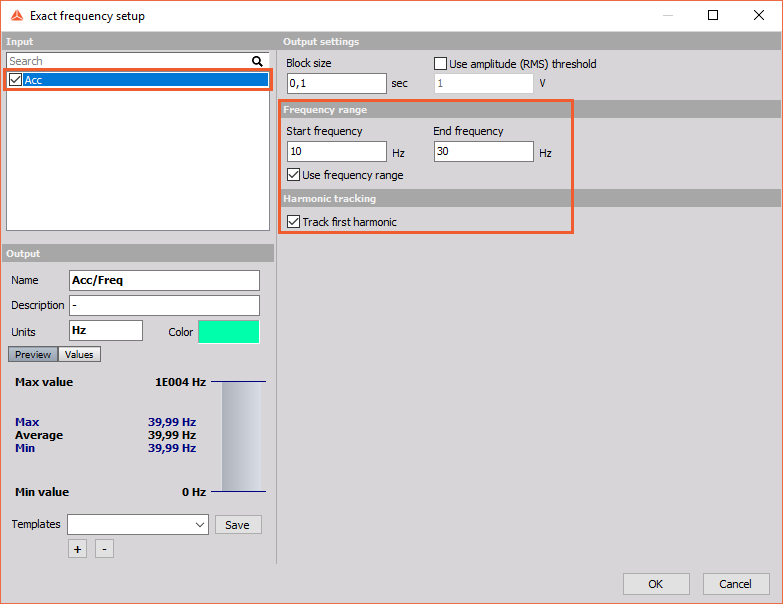Here is an example of order tracking just with the acceleration sensor.

When the machine is running, this is how the FFT looks like:To get the rotational frequency of motor, the Exact frequency math is used, with the option to Track the first harmonic.

This math calculates the frequency of the highest peak in FFT.The output channel shows me the frequency in Hz:In the OT setup, the RPM channel needs to be selected, for the frequency channyl use the channel from exact frequency math.During the measurement the FFT waterfall results can be observed on a 3D graph.